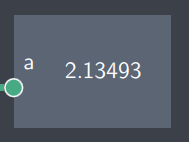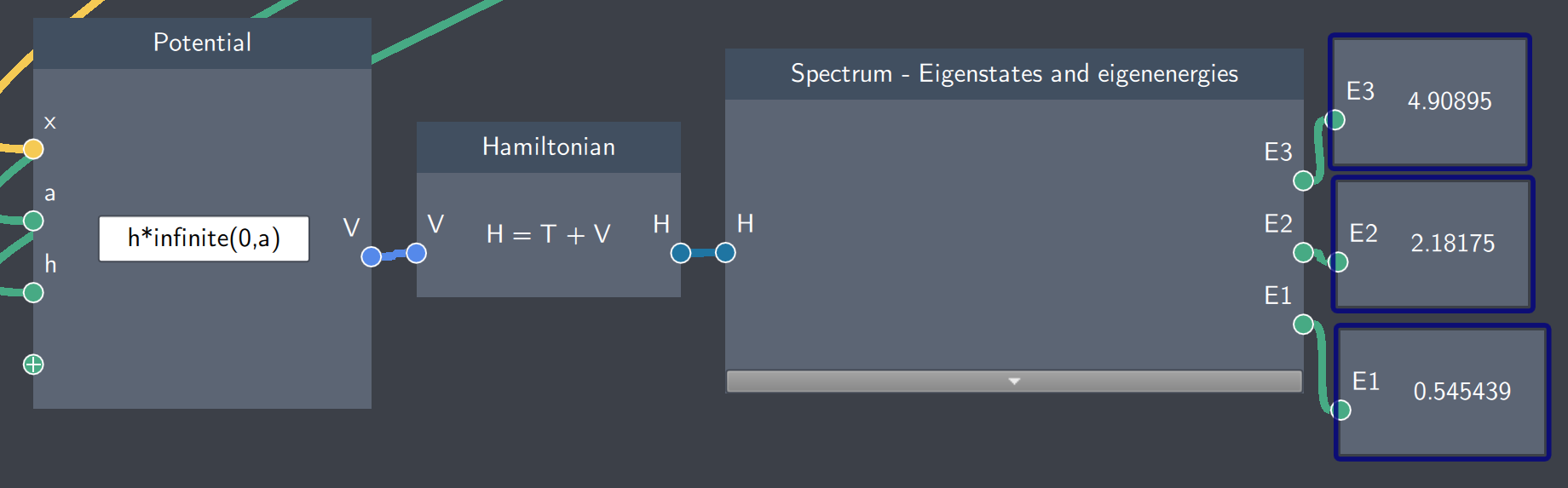# Scalar Output

## Description

This node displays a scalar quantity which is obtained as an output from another node.## Input

The node has the following input:

• Scalar (a): A scalar quantity output from another node.

## Content

The node displays the scalar quantity.

## Output

This node has no direct output.

## Example

In the example below, the spectrum of eigenenergies are calculated and the first three eigenvalues (E1, E2 and E3) are displayed in the Scalar Output nodes (blue-outlined boxes).